# GMAT Math : Circles

## Example Questions

### Example Question #7 : Dsq: Calculating The Length Of An Arc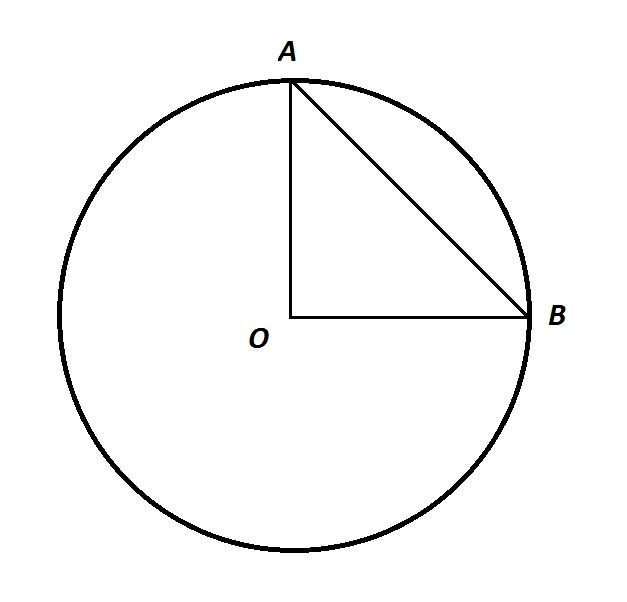Note: Figure NOT drawn to scale.

In the above figure,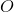is the center of the circle, and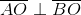. Give the length of arc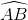.

Statement 1: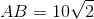.

Statement 2: The area of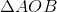is.

Statement 2 ALONE is sufficient to answer the question, but Statement 1 ALONE is NOT sufficient to answer the question.

EITHER statement ALONE is sufficient to answer the question.

BOTH statements TOGETHER are insufficient to answer the question.

BOTH statements TOGETHER are sufficient to answer the question, but NEITHER statement ALONE is sufficient to answer the question.

Statement 1 ALONE is sufficient to answer the question, but Statement 2 ALONE is NOT sufficient to answer the question.

EITHER statement ALONE is sufficient to answer the question.

Explanation:, makinga right triangle. Since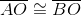, both segments being radii,is also a 45-45-90 triangle.

From Statement 1 alone, it can be determined by way of the 45-45-90 Theorem that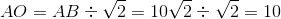.

From Statement 2 alone, since the area of a right triangle is half the product of its legs,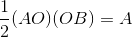Since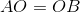and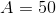,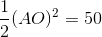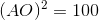From either statement alone, the radius of the circle can be calculated. From there, the circumference can be calculated, and the length of the arc can be found by multiplying the circumference by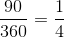### Example Question #1 : Dsq: Calculating The Angle Of A Sector

What time is it?

Statement 1: The minute hand and the hour hand are currently forming a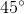angle.

Statement 2: The tip of the minute hand has traveled exactly eight inches since last leaving the 12 position.

EITHER statement ALONE is sufficient to answer the question.

Statement 1 ALONE is sufficient to answer the question, but Statement 2 ALONE is NOT sufficient to answer the question.

BOTH statements TOGETHER are sufficient to answer the question, but NEITHER statement ALONE is sufficient to answer the question.

Statement 2 ALONE is sufficient to answer the question, but Statement 1 ALONE is NOT sufficient to answer the question.

BOTH statements TOGETHER are insufficient to answer the question.

BOTH statements TOGETHER are insufficient to answer the question.

Explanation:

The two statements together are not enough unless you know the size of the minute hand; without this information, you cannot tell the angular position of the minute hand, so, even if you know the angle the hands are making, you do not know the position of the hour hand either.

### Example Question #1 : Dsq: Calculating The Angle Of A Sector

What time is it?

Statement 1: The minute hand and the hour hand form a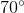angle.

Statement 2: The minute hand is exactly on the 8.

Statement 1 ALONE is sufficient to answer the question, but Statement 2 ALONE is NOT sufficient to answer the question.

BOTH statements TOGETHER are sufficient to answer the question, but NEITHER statement ALONE is sufficient to answer the question.

BOTH statements TOGETHER are insufficient to answer the question.

Statement 2 ALONE is sufficient to answer the question, but Statement 1 ALONE is NOT sufficient to answer the question.

EITHER statement ALONE is sufficient to answer the question.

BOTH statements TOGETHER are sufficient to answer the question, but NEITHER statement ALONE is sufficient to answer the question.

Explanation:

The first event happens numerous times over the course of twelve hours, so the first statement is not enough to deduce the time; all the second statement tells you is that it is forty minutes after an hour (12:40, 1:40, etc.)

Suppose we put the two statements together. It is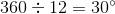from one number to the next, so the 8:00 position is the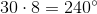position. If the hour hand makes awith the minute hand, then the hour hand is either at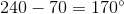or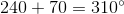. Since forty minutes is two-thirds of an hour, however, the hour hand must be two-thirds of the way from one number to the next.

Case 1: If the hour hand is at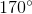, then it is at the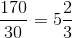position - in other words, two-thirds of the way from the 5 to the 6. This is consistent with our conditions.

Case 2: If the hour hand is at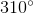, then it is at the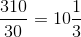position - in other words, one-third of the way from the 10 to the 11. This is inconsistent with our conditions.

Therefore, only the first case is possible, and if we are given both statements, we know it is 5:40.

### Example Question #3 : Dsq: Calculating The Angle Of A Sector

What time is it?

Statement 1: The minute hand and the hour hand are currently forming a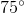angle.

Statement 2: The minute hand is on the 6.

Statement 2 ALONE is sufficient to answer the question, but Statement 1 ALONE is NOT sufficient to answer the question.

BOTH statements TOGETHER are insufficient to answer the question.

EITHER statement ALONE is sufficient to answer the question.

BOTH statements TOGETHER are sufficient to answer the question, but NEITHER statement ALONE is sufficient to answer the question.

Statement 1 ALONE is sufficient to answer the question, but Statement 2 ALONE is NOT sufficient to answer the question.

BOTH statements TOGETHER are insufficient to answer the question.

Explanation:

Since there are twelve numbers on the clock, the angular measure from one number to the next is; this meansrepresents two and a half number positions.

Suppose we know both statements. Since the minute hand is on the 6, the hour hand is either midway between the 3 and the 4, or midway between the 8 and the 9. Both scenarios are possible, as they correspond to 3:30 and 8:30, respectively, so the question is not answered even if we know both statements.

### Example Question #1 : Dsq: Calculating The Angle Of A Sector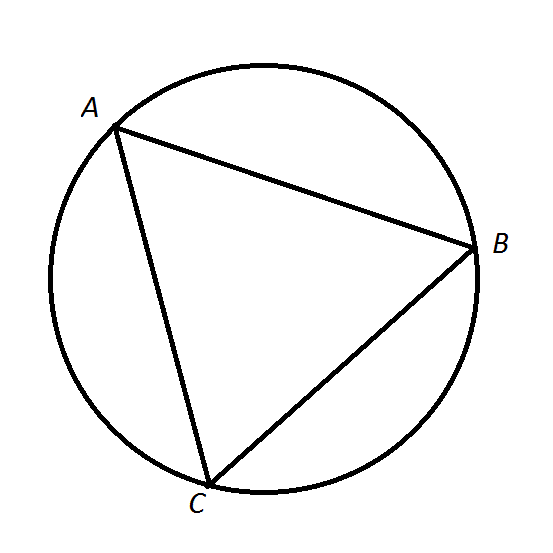Note: Figure NOT drawn to scale.

Refer to the above figure. What is the degree measure of?

Statement 1: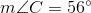.

Statement 2: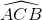measures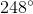.

EITHER statement ALONE is sufficient to answer the question.

BOTH statements TOGETHER are insufficient to answer the question.

Statement 2 ALONE is sufficient to answer the question, but Statement 1 ALONE is NOT sufficient to answer the question.

BOTH statements TOGETHER are sufficient to answer the question, but NEITHER statement ALONE is sufficient to answer the question.

Statement 1 ALONE is sufficient to answer the question, but Statement 2 ALONE is NOT sufficient to answer the question.

EITHER statement ALONE is sufficient to answer the question.

Explanation:

From Statement 1, the measure of the arc can be determined by doubling the measure of the intercepting angle, which is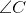. From Statement 2, the measure of the arc can be calculated by subtracting from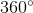the degree measure of the corresponding major arc, which is### Example Question #1 : Dsq: Calculating The Angle Of A Sector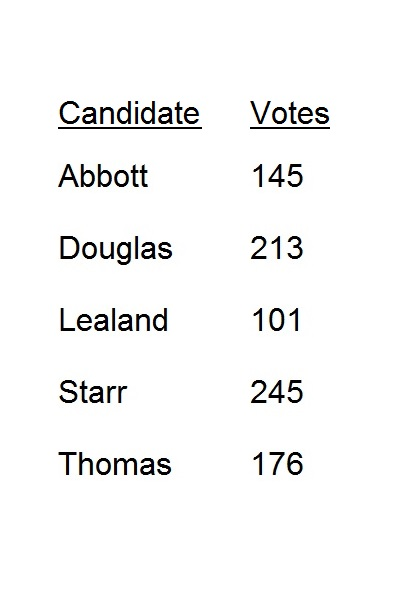Above are the results of the election for student body president that Greg is about to publish in the school newspaper. At the last minute, his friend Melissa stops him and reminds him that there was a sixth candidate, Wilson, who got 202 votes.

Greg's article includes a circle graph that he will now have to change to reflect this corrected information. By how many degrees will the angle measure of the sector representing Douglas decrease (nearest whole degree)?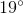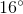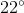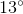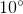Explanation:

According to Greg's erroneous information, the number of people who voted was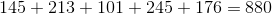.

213 voted for Douglas, meaning that his sector will have degree measure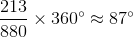Based on the new information,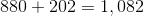people voted.

213 voted for Douglas, meaning that his sector will have degree measure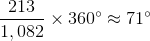The reduction in degree measure will be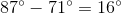.

### Example Question #1 : Dsq: Calculating The Angle Of A SectorAfter a student body election, Henry is constructing a circle graph to represent the above voter count.

What will be the measure of the central angle of the sector representing Thomas (nearest whole number)?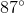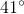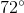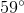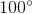Explanation:

The number of people who voted:176 people voted for Starr, so the sector representing Starr will have measure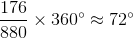### Example Question #611 : Data Sufficiency Questions

Circle A has radius six times that of Circle B; a sector of Circle A with angle measure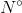has the same area as Circle B. Evaluate.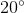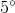Explanation:

Letbe the radius of Circle B. Then Circle A has radius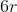and, subsequently, area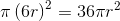. Since the area of Circle B is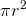, the area of Circle A is 36 times that of Circle B.

The given sector of Circle A has the same area as Circle B, so the sector is one thirty-sixth of the circle. That makes the angle measure of the sector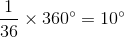### Example Question #61 : Circles

The radius of Circle A is equal to the sidelength of Square B. A sector of Circle A has the same area as Square B. Which of the following is the degree measure of this sector?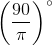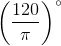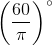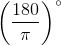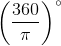Explanation:

The radius of Circle A and the length of a side of the square are the same - we will call each. The area of the circle is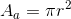; that of the square is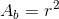. Therefore, a sector of the circle with area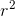will be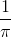of the circle, which is a sector of measure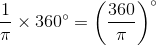### Example Question #1 : Dsq: Calculating The Angle Of A SectorAfter a student body election, Henry is constructing a circle graph to represent the above voter count.

What will be the measure of the central angle of the sector representing Starr (nearest whole number)?Explanation:

The number of people who voted:245 people voted for Starr, so the sector representing Starr will have measure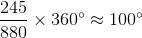### All GMAT Math Resources